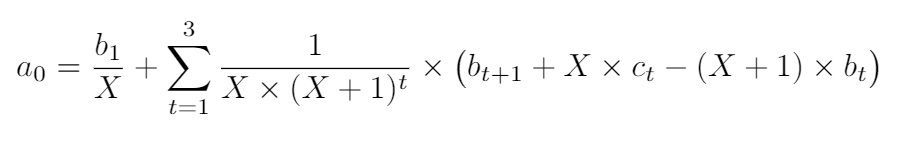## How to estimate solution of an autoregressive equation of other known variables in timeseries data?

Hello,

I am a novice user and my data is a time series that consists of different companies with unique id, date, stock price, EPS and dividend. For the sake of simplicity I made a sample as below:

``````data have;
input id date a b c;
datalines;
1 201001 56 7  1
1 201002 58 6  2
1 201003 54 7  1
1 201004 55 5  3
2 201001 80 10 5
2 201002 81 11 3
2 201003 83 12 2
3 201001 20 3  1
3 201002 22 2  1
/* etc. */
;  ``````

I'm trying to estimate/create the variable X for every obsevation that is the solution of an autoregressive equation of a, b and c as follows:In this example the equation envolves values going up to 3 periods into the future, so I suppose I can use brute force method and create all 3 future values of b and c. However if I want to examine further, say 36 or 48 periods, things will get very messy. Even if this is done, the equation is still a (very long) cubic equation, which to be honest at this point I'm not sure how to estimate.

I would really appreciate any suggestions here. Thank you very much in advance.

Best.

Discussion stats
• 0 replies
• 253 views
• 0 likes
• 1 in conversation